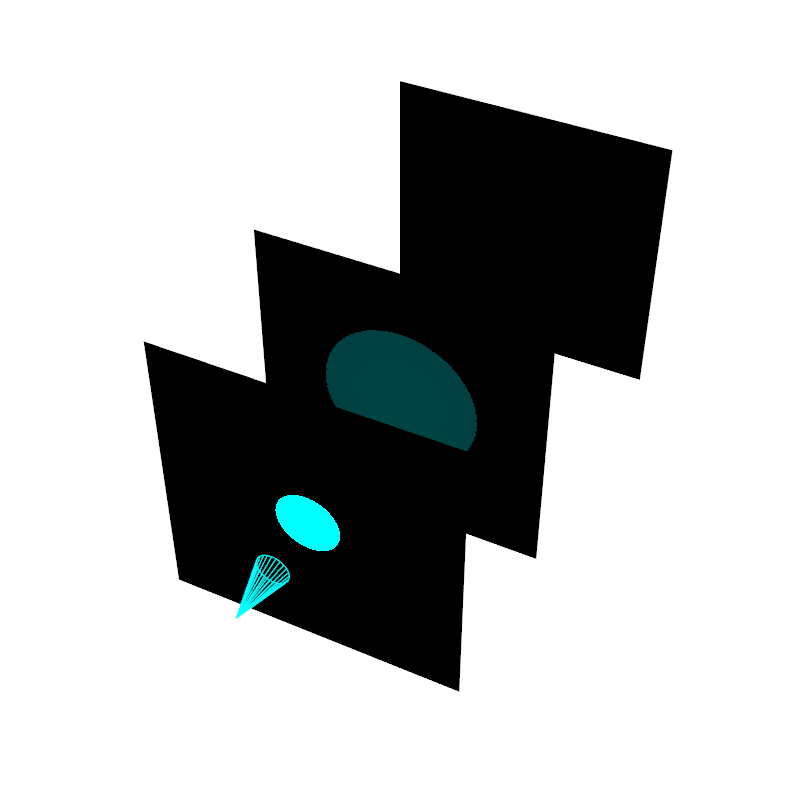Demonstrate the usage of lights and shadows in PyVista.

```# sphinx_gallery_thumbnail_number = 2
import pyvista
from pyvista import examples
import numpy as np

mesh.rotate_x(90)
mesh.rotate_z(120)
```

Using two lights, plot the Stanford Dragon with shadows.

Note

VTK has known issues when rendering shadows on certain window sizes. Be prepared to experiment with the `window_size` parameter. An initial window size of `(1000, 1000)` seems to work well, which can be manually resized without issue.

```light1 = pyvista.Light(position=(0, 0.2, 1.0),
focal_point=(0, 0, 0),
color=[1, 1.0, 0.9843, 1],  # Color temp. 5400 K
intensity=0.3)

light2 = pyvista.Light(position=(0, 1.0, 1.0),
focal_point=(0, 0, 0),
color=[1, 0.83921, 0.6666, 1],  # Color temp. 2850 K
intensity=1)

# Add a thin box below the mesh
bounds = mesh.bounds
rnge = (bounds - bounds,
bounds - bounds,
bounds - bounds)

expand = 1.0
height = rnge * 0.05
center = np.array(mesh.center)
center -= [0, 0, mesh.center - bounds + height/2]

width = rnge*(1 + expand)
length = rnge*(1 + expand)
base_mesh = pyvista.Cube(center,
width,
length,
height)

# rotate base and mesh to get a better view
base_mesh.rotate_z(30)
mesh.rotate_z(30)

# create the plotter with custom lighting
pl = pyvista.Plotter(lighting=None, window_size=(800, 800))
pl.camera.zoom(1.5)
pl.show()
```Show light penetrating several planes. Adjust the light intensity and the `shadow_attenuation` to change how many planes the light can go through.

```plotter = pyvista.Plotter(lighting=None, window_size=(800, 800))

for plane_y in [2, 5, 10]:
screen = pyvista.Plane(center=(0, plane_y, 0), direction=(0, 1, 0),
i_size=5, j_size=5)

light = pyvista.Light(position=(0, 0, 0), focal_point=(0, 1, 0),
color='cyan', intensity=15, positional=True,
cone_angle=15, attenuation_values=(2, 0, 0))
light.show_actor()

plotter.view_vector((1, -2, 2))
plotter.show()
```Here, we use a lower shadow_attenuation value to demonstrate how the light can travel through more planes.

```plotter = pyvista.Plotter(lighting=None, window_size=(800, 800))

for plane_y in [2, 5, 10]:
screen = pyvista.Plane(center=(0, plane_y, 0), direction=(0, 1, 0),
i_size=5, j_size=5)

light = pyvista.Light(position=(0, 0, 0), focal_point=(0, 1, 0),
color='cyan', intensity=15, cone_angle=15,